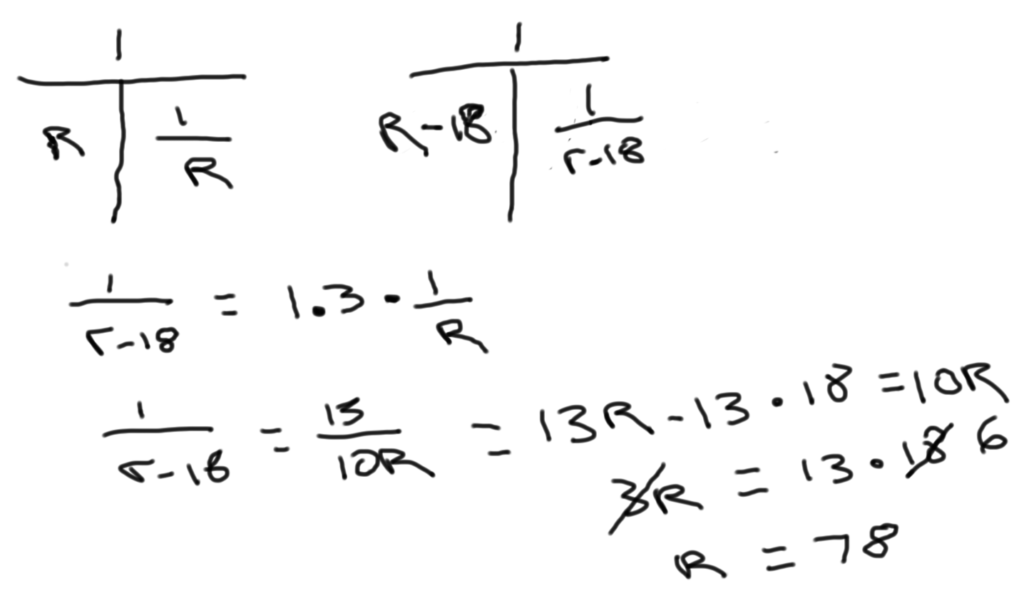# GMAT Question of the Day - PS Work/Rate - May 2nd, 2014

The express train would have taken 30% longer to get from Station K to Station M if it had been traveling 18 miles per hour slower. What was the express train's actual average speed, in miles per hour, from Station K to Station M?

A. 78

B. 82

C. 84

D. 85

E. 93

Here's an Official GMAT question on which we also use the T method to organize the information: A boat traveled upstream a distance of 90 miles at an average speed of (v-3) miles per hour and then traveled the same distance downstream at an average speed of (v+3) miles per hourDistance, Work and Rate - Average Rate 1

Distance, Work and Rate - Average Rate 2

For more GMAT distance, work, and rate practice visit GMAT Question of the Day.

# CONTACT

Atlantic GMAT

405 East 51st St.

NY, NY 10022

(347) 669-3545

info@AtlanticGMAT.com